# DC Pandey Solutions: Electrostatics - 1 Notes | Study Physics Class 12 - NEET

## NEET: DC Pandey Solutions: Electrostatics - 1 Notes | Study Physics Class 12 - NEET

The document DC Pandey Solutions: Electrostatics - 1 Notes | Study Physics Class 12 - NEET is a part of the NEET Course Physics Class 12.
All you need of NEET at this link: NEET

Introductory Exercise 21.1

Q.1. Is attraction a true test of electrification?

Ans. Due to induction effect, a charged body can attract a neutral body as shown below.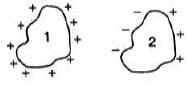Body-1 is positively charged and body-2 is neutral. But we can see that due to distance factor attraction is more than the repulsion.

Q.2. What is the total charge, in coulombs, of all the electrons in a three-gram mole of hydrogen atoms?

Ans. Number of atoms in 3 gram-mole of hydrogen atom = number of electrons in it
= 3N0 = (3 × 6.02 × 1023) where
∴ Total charge
= - (1.6 × 10-19) (3 × 6.02 × 1023)
= -2.89 × 105C

Introductory Exercise 21.2

Q.1. The mass of an electron is 9.11 × 10-31 kg, that of a proton is 1.67 × 10-27 kg. Find the ratio Fe/Fg of the electric force and the gravitational force exerted by the proton on the electron,
Ans.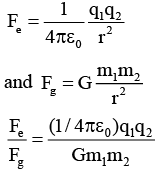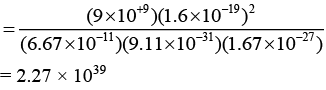Q.2. Find the dimensions and units of ∈0.
Ans.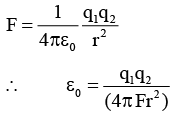Units and dimensions can be found by above equation.

Q.3. Three point charges q are placed at three vertices of an equilateral triangle of side a. Find magnitude of electric force on any charge due to the other two.
Ans.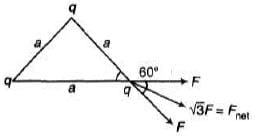F = Force between can two point charges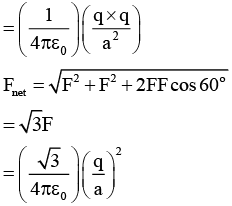Q.4. Three point charges each of value +q are placed on three vertices of a square of side a metre. What is the magnitude of the force on a point charge of value-q coulomb placed at the centre of the square?
Ans.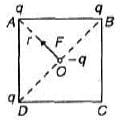Net force on -q from the charges at B and D is zero. So net force on - q is only due to the charge at A.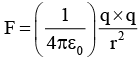where,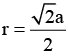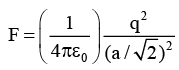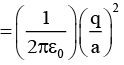Q.5. Is there any lower limit to the electric force between two particles placed at a certain distance?
Ans.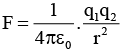(q1)min = (q2)min
∴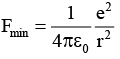Q.6. The electric force on a charge qdue to q2 is (4 i - 3 j) N. What is the force on qdue to q1?

Ans. Two forces are equal and opposite.

Introductory Exercise 21.3

Q.1: The electric field of a point charge is uniform. Is it true or false?

Ans. Electric field lines are not parallel and equidistant.

Q.2. Electric field lines are shown in Fig.. State whether the electric potential is greater at A or B.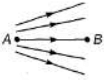Ans. Electric lines flow higher potential to lower potential.
VA > VB

Q.3. A charged particle always move in the direct ion of electric field. Is this statement true or false?

Ans. If charged particle is positive, and at rest. Electric field lines are straight then only it will move in the direction of electric field.

Q.4. What is the charge per unit area in C/m2, of an infinite plane sheet of charge if the electric field produced by the sheet of charge has magnitude 3.0 N/C?

Ans.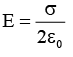∴ σ= (2E ε0)
= 2 × 3.0 × 8.86 × 10-12 = 5.31 × 10-11 C/m2

Q.5. The figure shows some of the electric field lines due to three-point charges q1, q2, and q3 of equal magnitude. What are the signs of each of the three charges?

Ans. Electric field lines start from positive charge and terminate on a negative charge.

Q.6. Four particles each having a charge q, are placed on the four vertices of a regular pentagon. The distance of each corner from the centre is a. Find the electric field at the centre of the pentagon.

Ans. In case of five charges at five vert ices o f regular pentagon net electric field at centre is zero. Because five vectors of equal magnitudes from a closed regular pentagon at shown in figure (i).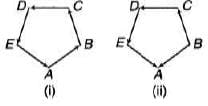Where one charge is removed. Then one vector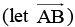is deceased hence the net resultant is equal to magnitude of one vector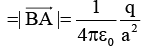Q.7. A circular wire loop of radius R carries a total charge q distributed uniformly over its length. A small length x( << R) of the wire is cut off. Find the electric field at the centre due to the remaining wire.
Ans.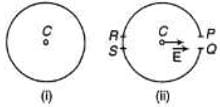If loop is complete, then net electric field at centre C is zero. Because equal and opposite pair of electric field vectors are canceled.
If PQ portion is removed as shown in figure, then electric field due to portion RS is not cancelled. Hence electric field is only due to the option RS.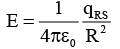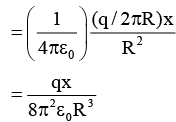Q.8. A charge q = -2.0 μC is placed at origin. Find the electric field at (3m, 4m, 0).
Ans.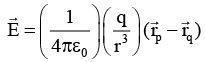Here r =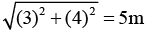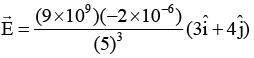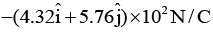The document DC Pandey Solutions: Electrostatics - 1 Notes | Study Physics Class 12 - NEET is a part of the NEET Course Physics Class 12.
All you need of NEET at this link: NEETUse Code STAYHOME200 and get INR 200 additional OFF

## Physics Class 12

134 videos|388 docs|213 tests

### How to Prepare for NEET

Read our guide to prepare for NEET which is created by Toppers & the best Teachers

Track your progress, build streaks, highlight & save important lessons and more!

,

,

,

,

,

,

,

,

,

,

,

,

,

,

,

,

,

,

,

,

,

;